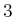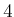#### Other extensions

Several ideas from this section can be generalized to produce other navigation functions. One disadvantage of the methods discussed so far is that undesirable staircase motions (as shown in Figure 7.40) are produced. If the 2-neighborhood, as defined in (5.38), is used to define the action spaces, then the motions will generally be shorter. Dial's algorithm can be applied to efficiently compute an optimal navigation function in this case.

A grid approximation can be made to higher dimensional configuration spaces. Since a high resolution is needed, however, it is practical only for a few dimensions (e.g.,or). If the 1-neighborhood is used, then wavefront propagation can be easily applied to compute navigation functions. Dial's algorithm can be adapted for general-neighborhoods.

Constructing navigation functions over grids may provide a practical solution in many applications. In other cases it may be unacceptable that staircase motions occur. In many cases, it may not even be possible to compute the navigation function quickly enough. Factors that influence this problem are 1) very high accuracy, and a hence high-resolution grid may be necessary; 2) the dimension of the configuration space may be high; and 3) the environment may be frequently changing, and a real-time response is required. To address these issues, it is appealing to abandon grid approximations. This will require defining potential functions and velocities directly on the configuration space. Section 8.3 presents the background mathematical concepts to make this transition.

Steven M LaValle 2020-08-14